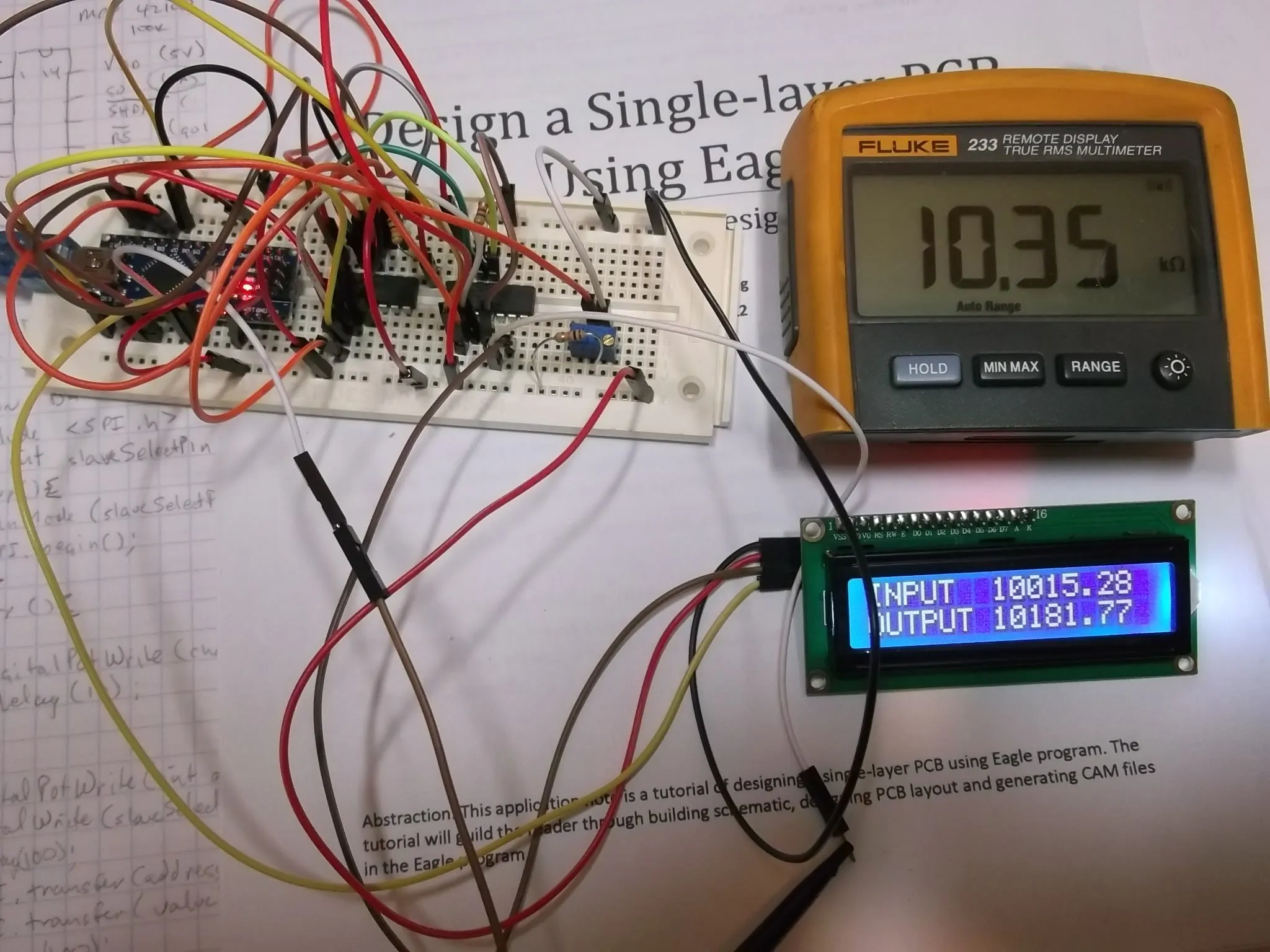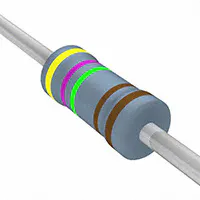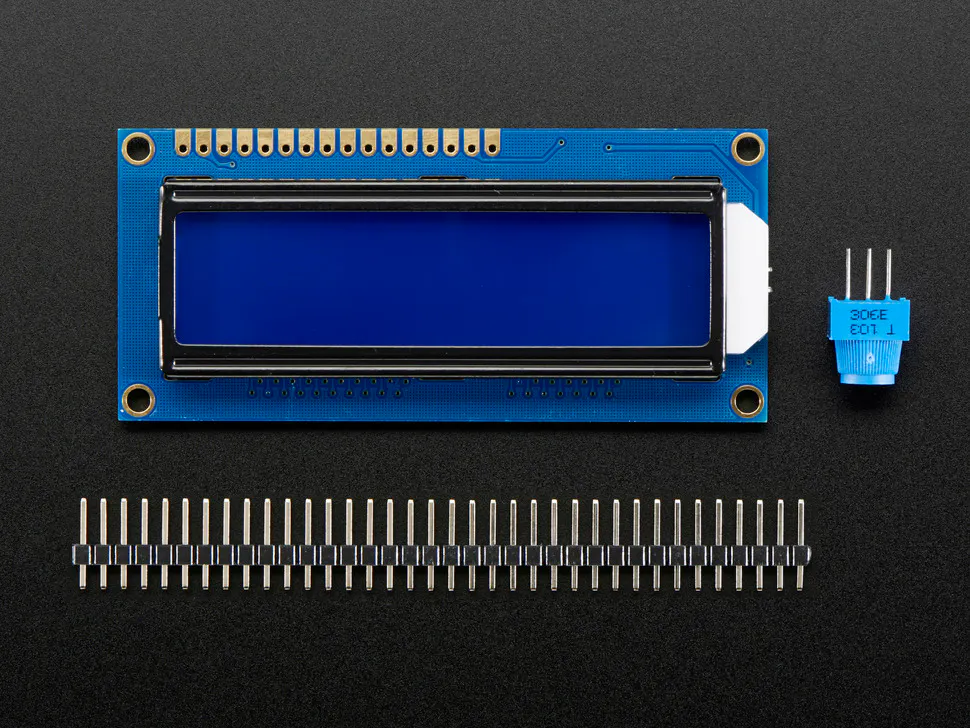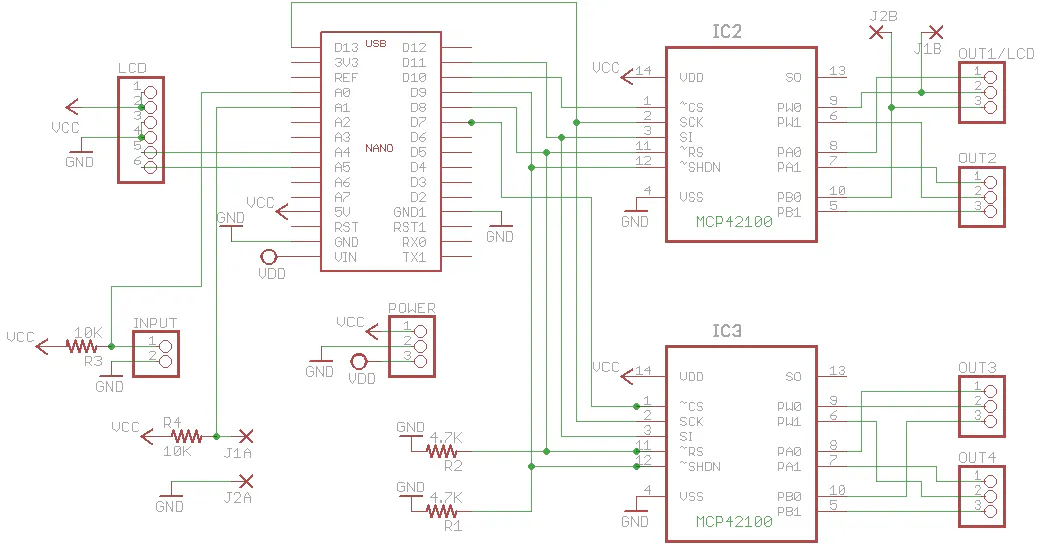# Resistor Duplicator

This circuit allows for one thermistor input and will output up to four similar values using digital pots.

IntermediateProtip1 hour1,424## Things used in this project

### Hardware componentsArduino Nano R3
×1
 MCP42100
×1Resistor 4.75k ohm
×2Resistor 10k ohm
×2Adafruit Standard LCD - 16x2 White on Blue
×1
 lcd backpack for I2C communication
×1

### Software apps and online servicesArduino IDE

## Schematics

### Resistor Multiplier

This is the scheme I used. Note that there are jumpers for using the LCD or the output. Connect jumpers for output value in readout.## Code

### Resistor Multiplier

Arduino
For operating two MCP42100 digipots and an LCD
```/*
This code used to control the digital
potentiometer MCP42100 connected to arduino board

SCLK >>D13
DI >>> D11
CS >>> D10   chip IC2
SHDN >>D9
RS >>> D8
CS >>> D7   chip IC3
input >>> A0
output >>> A1
SDA >>> A4
SCL >>> A5

*/

#include <Wire.h>
#include <LiquidCrystal_I2C.h>
#include <SPI.h>
byte addressPot0 = 0b00010001; // last two bits 01 is Pot 0
byte addressPot1 = 0b00010010; // last two bits 10 is Pot 1
byte addressPot0and1 = 0b00010011; // last two bits 11 is Pot 0 and 1
byte CS1 = 10; //first chip select
byte CS2 = 7;  //second chip select
byte SHDN = 9; //chip shutdown is pin 9 use 4700 ohm for pulldown
byte RS = 8;  //chip reset is pin 8 use 4700 ohm for pulldown
byte thermister = 0;  //thermister input on A0
byte reference = 1;  //second pot input for lcd

float PotRatio = 390.625; //100000/256
float Vout=0;
float Vin=1024;
float R1=9860;  //measured resistance value for input R3
float R2=9830;  //measured resistance value for output R4
float out1=0;
float out2=0;
int out3=0;
int out4=0;
float Voutb=0;
float out1b=0;
float out2b=0;
int out3b=0;
LiquidCrystal_I2C lcd(0x3F,16,2);  // for 16 x 2

void setup()
{
pinMode (CS1,OUTPUT);  //when high chip reads data
pinMode (CS2,OUTPUT);  //when high chip reads data
pinMode (SHDN, OUTPUT);  //when high chip reads data
pinMode (RS,OUTPUT);  //when high chip reads data
pinMode (thermister, INPUT);
pinMode (reference, INPUT);
digitalWrite (SHDN, HIGH);  //power up chip (high)
digitalWrite (RS, HIGH);  //power on reset (low)

SPI.begin();

digitalWrite(SHDN, LOW);  //power off
digitalWrite(SHDN,HIGH);  //power on
digitalWrite(RS,LOW); //power on reset
delay(100);
digitalWrite(RS, HIGH);  //power reset
delay (2000);

lcd.init();

lcd.backlight();
lcd.setCursor(0,0);
lcd.print("INPUT");
lcd.setCursor(0,1);
lcd.print("OUTPUT");
}

void loop()
{
//PotHighAndLow_mt(addressPot0);  // change values on pot 0
//PotHighAndLow_mt(addressPot1);  // change values on pot 1
PotHighAndLow_mt(addressPot0and1);  // change values on both pots
}

{
out1=(Vout/((Vin-Vout)/R1));
out2=out1/PotRatio;
out3=round(out2);
out4=round(out1);
delay(10);
out1b=(Voutb/((Vin-Voutb)/R2));
}

{
digitalWrite(CS1, LOW);  //set chip IC2 active
lcd.setCursor(7,0);
lcd.print(out1);
lcd.setCursor(7,1);
lcd.print(out1b);
SPI.transfer(value);
delay(10);
digitalWrite(CS1, HIGH);  //set chip IC2 inactive
digitalWrite(CS2, LOW);  // set chip IC3 active
SPI.transfer(value);
delay(10);
digitalWrite(CS2, HIGH);  //set chip IC3 inactive
}
```

## Credits

### Kevin Strain

3 projects • 11 followers
Nothing special